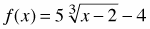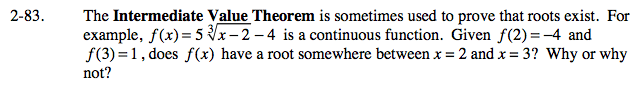### Home > CALC > Chapter 2 > Lesson 2.2.3 > Problem2-83

2-83.

The Intermediate Value Theorem is sometimes used to prove that roots exist. For example,is a continuous function. Given f(2) = −4 and f(3) = 1, does f(x) have a root somewhere between x = 2 and x = 3? Why or why not? Homework Help ✎Is it possible to move from y = –4 to y = 1 without passing through y = 0?

Use the eTool below to view the graph of the functions.
Click on the link to the right to view the full version of the eTool: Calc 2-82 HW eTool